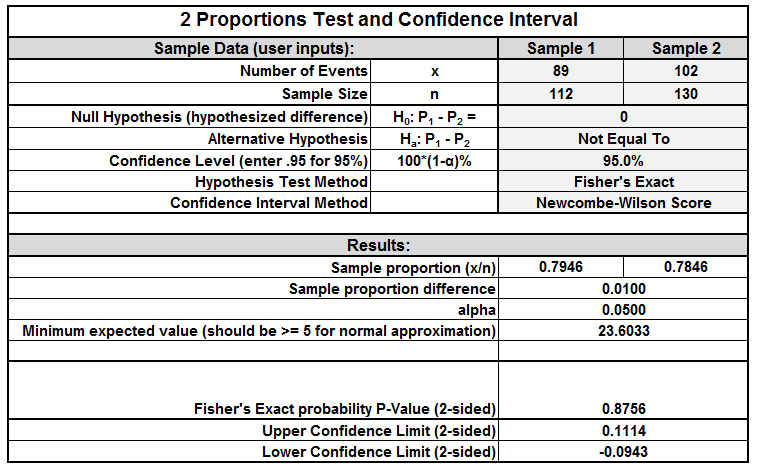# Two Sample Proportion Test with SigmaXL

### What is the Two Sample Proportion Test with SigmaXL?

The two sample proportion test with SigmaXL is a hypothesis test to compare the proportions of one certain event occurring in two populations following the binomial distribution.

• Null Hypothesis(H0): p1 = p2
• Alternative Hypothesis(Ha): p1 ≠ p2

### Two Sample Proportion Test Assumptions

• The sample data drawn from the populations of interest are unbiased and representative.
• There are only two possible outcomes in each trial for both populations: success/failure, yes/no, and defective/non-defective etc.
• The underlying distributions of both populations are binomial distribution.
• When np ≥ 5 and np(1 – p) ≥ 5, the binomial distribution can be approximated by the normal distribution.

When |Zcalc| is smaller than Zcrit, we fail to reject the null hypothesis.

### Run a Two Sample Proportion Test with SigmaXL

Case study: We are interested in comparing the exam pass rates of a high school in March and April using a nonparametric (i.e. distribution-free) hypothesis test: two sample proportion test.
Data File: “Two Sample Proportion” tab in “Sample Data.xlsx”

• Null Hypothesis (H0): pMarch = pApril
• Alternative Hypothesis (Ha): pMarch ≠ pApril

#### Steps to run a two sample proportion test in SigmaXL:

1. Click SigmaXL -> Statistical Tools -> 2 Proportions Test & Confidence Interval
2. A new tab named “2 Proportions Test and CI” appears automatically.
3. Enter “89” in the yellow box of “Number of Events” in Sample #1 column
4. Enter “112” in the yellow box of “Sample Size in Sample #1 column.
5. Enter “102” in the yellow box of “Number of Events” in Sample #2 column.
6. Enter “130” in the yellow box of “Sample Size” in Sample #2 column.
7. Select “Fisher’s Exact” as the testing method.Model summary: The p-value of the two-sample proportion test is 0.8756, greater than the alpha level (0.05), and we fail to reject the null hypothesis. We conclude that the exam pass rates of the high school in March and April are not statistically different.